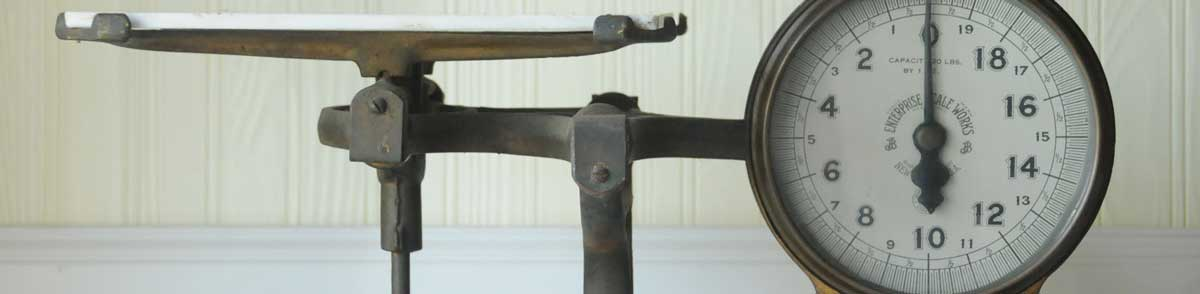# Pounds to Calories Conversion

Enter the weight in pounds below to get the value converted to calories.

lb
oz
Results in Calories:1 lb = 3,500 kcal
Do you want to convert calories to pounds?

## How to Convert Pounds to CaloriesTo convert a pound measurement to a calorie measurement, multiply the weight by the conversion ratio.

Since one pound of body fat is equal to 3,500 calories, you can use this simple formula to convert:

calories = pounds × 3,500

The weight in calories is equal to the pounds multiplied by 3,500.

For example, here's how to convert 5 pounds to calories using the formula above.
5 lb = (5 × 3,500) = 17,500 kcal
So, to lose 5 pounds, you would need to burn 17,500 additional calories.

### How Many Calories are in a Pound of Body Fat?

There are 3,500 calories in a pound of body fat, which is why we use this value in the formula above.

1 lb = 3,500 kcal

Pounds are a unit of weight, and calories are a unit of energy. Keep reading to learn more about each unit of measure.

## Pounds

One pound is defined as a unit of mass/weight equal to 16 ounces, or 0.45359237 kilograms. One pound is equal to 7,000 grains in the avoirdupois or apothecaries' systems.

The pound is a US customary and imperial unit of weight. A pound is sometimes also referred to as a common ounce. Pounds can be abbreviated as lb, and are also sometimes abbreviated as lbs, lbm, or #. For example, 1 pound can be written as 1 lb, 1 lbs, 1 lbm, or 1 #.

## Calories

Converting calories is actually a conversion of energy burned by the body performing work rather than actual weight. When measuring the energy content in foods, the large calorie, or kilocalorie, is used, but it's often still just called a "calorie."

One calorie (large calorie) is equal to the heat needed to raise the temperature of one kilogram of water by 1 degree Celsius. You'll need to burn 3,500 calories more than you eat to lose one pound of weight.

Calories can be abbreviated as kcal; for example, 1 calorie can be written as 1 kcal.

## Pound to Calorie Conversion Table

Pound measurements converted to calories
Pounds Calories
0.001 lb 3.5 kcal
0.002 lb 7 kcal
0.003 lb 10.5 kcal
0.004 lb 14 kcal
0.005 lb 17.5 kcal
0.006 lb 21 kcal
0.007 lb 24.5 kcal
0.008 lb 28 kcal
0.009 lb 31.5 kcal
0.01 lb 35 kcal
0.02 lb 70 kcal
0.03 lb 105 kcal
0.04 lb 140 kcal
0.05 lb 175 kcal
0.06 lb 210 kcal
0.07 lb 245 kcal
0.08 lb 280 kcal
0.09 lb 315 kcal
0.1 lb 350 kcal
0.2 lb 700 kcal
0.3 lb 1,050 kcal
0.4 lb 1,400 kcal
0.5 lb 1,750 kcal
0.6 lb 2,100 kcal
0.7 lb 2,450 kcal
0.8 lb 2,800 kcal
0.9 lb 3,150 kcal
1 lb 3,500 kcal

## References

1. Encyclopædia Britannica, Avoirdupois weight, https://www.britannica.com/science/avoirdupois-weight
2. Brazier, Yvette, How many calories do you need?, Medical News Today, https://www.medicalnewstoday.com/articles/263028
3. U.S. Department of Agriculture, I want to lose a pound of weight. How many calories do I need to burn?, https://www.nal.usda.gov/fnic/i-want-lose-pound-weight-how-many-calories-do-i-need-burn